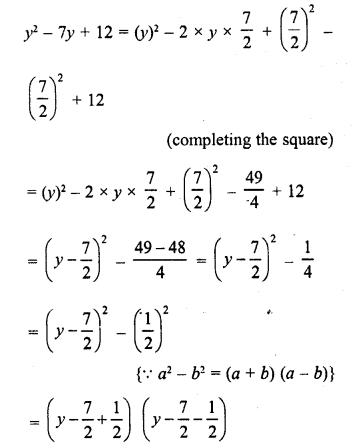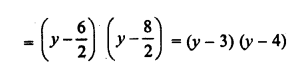## RD Sharma Class 8 Solutions Chapter 7 Factorizations Ex 7.9

These Solutions are part of RD Sharma Class 8 Solutions. Here we have given RD Sharma Class 8 Solutions Chapter 7 Factorizations Ex 7.9

Other Exercises

Factorize each of the following quadratic polynomials by using the method of completing the square.
Question 1.
p2 + 6p + 8
Solution:
p2 + 6p + 8
= p2 + 2 x p x 3 + 32 – 32 + 8   (completing the square)
= (p2 + 6p + 32) – 1
= (p + 3)2 – 12
= (P + 3)2 – (1)2       { ∵ a2 + b2  = (a+b) (a-b)}
= (p +3+1) (p + 3 -1)
= (p+4) (p+ 2)

Question 2.
q2 – 10q + 21
Solution:
q2 – 10q + 21
= (q)2 – 2 x q x 5 + (5)2 – (5)2 + 21   (completing the square)
= (q)2 – 2 x q x 5 + (5)2 -25+21
= (q)2-2 x q x 5 + (5)2 – 25 +21
= (q)2-2 x q x 5 + (5)2 – 4
= (q – 5)2 – (2)     {∵ a2 – b2 = (a + b) (a – b)}
= (q- 5 + 2) (q-5-2)
=(q- 3) (q-7)

Question 3.
4y2 + 12y + 5
Solution:
4y+12y + 5
= (2y)2 + 2 x 2y x 3 + (3)2 – (3)2 + 5    (completing the square)
= (2y + 3)2 – 9 + 5
= (2y + 3)2 – 4
= (2y + 3)2-(2)2   {∵ a2 – b2 = (a + b) (a – b)}
= (2y + 3 + 2) (2y + 3 – 2)
= (2y + 5) (2y+ 1)

Question 4.
p2 + 6p- 16
Solution:
p2 + 6p – 16
= (p)2 + 2 x  p x 3 + (3)2 – (3)2 – 16    (completing the square)
= (p)2 + 2 x p x 3 + (3)2 – 9 – 16
= (p + 3)2 – 25
= (p + 3)2 – (5)2     {∵ a2 -b2 = {a + b) (a – b)}
= (p + 3 + 5)(p + 3-5)
= (p + 8) (p – 2)

Question 5.
x2 + 12x + 20
Solution:
x2 + 12x + 20
= (x)2 + 2 x x x 6 + (6)2 – (6)2 + 20   (completing the square)
= (x)2 + 2 x x x6 + (6)2 -36 + 20
= (x + 6)2 -16
= (x + 6)2 – (4)2   {∵ a2 – b2 = (a + b) (a – b)}
= (x + 6 + 4) (x + 6 – 4)
= (x + 10) (x + 2)

Question 6.
a2 – 14a – 51
Solution:
a2 – 14a-51
= (a)2 – 2 x x 7 + (7)2 – (7)2 – 51       (completing the square)
= (a)2 – 2 x a x 7 + (7)2 – 49 – 51
= (a – 7)2 – 100
= (a – 7)2 – (10)2    {∵  a2 – b2 = (a + b) (a – b)}
= (a – 7 + 10) (a – 7 – 10)
= (a + 3) (a – 17)

Question 7.
a2 + 2a – 3
Solution:
a2 + 2a – 3
= (a)2 + 2 x a x 1 + (1)2 – (1)2 – 3   (completing the square)
= (a)2 + 2 x a x 1 + (1)2 – 1 – 3
= (a + 1)2 – 4
= (a + 1)2 – (2){∵ a2 – b2 = (a + b) (a – b)}
= (a + 1 + 2) (a + 1 – 2)
= (a + 3) (a – 1)

Question 8.
4x2 – 12x + 5
Solution:
4x2 – 12x + 5
= (2x)2 – 2 x 2x x 3 + (3)2 – (3)2 + 5  (completing the square)
= (2x)2 – 2 x 2x x 3 + (3)2 -9 + 5
= (2x – 3)2 – 4
= (2x – 3)2 – (2)2
{∵ a2b2 = (a + b) (a – b)}
=
(2x – 3 + 2) (2x – 3 – 2)
= (2x – 1) (2x – 5)

Question 9.
y2 – 7y + 12
Solution:Question 10.
z2-4z-12
Solution:
z2 – 4z – 12
= (z)2 – 2 z x 2 + (2)2 – (2)2 – 12  (completing the square)
= (z)2 – 2 x z x 2 + (2)2 – 4 – 12
= (z-2)2-16
= (z-2)2-(4)2   {∵ a2 – b2 = (a + b) (a – b)}
= (z – 2 + 4) (z – 2 – 4)
= (z + 2)(z-6)

Hope given RD Sharma Class 8 Solutions Chapter 7 Factorizations Ex 7.9 are helpful to complete your math homework.

If you have any doubts, please comment below. Learn Insta try to provide online math tutoring for you.